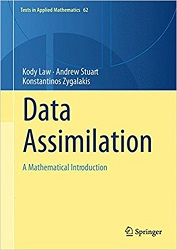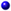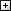Processing ......FreeComputerBooks.com Free Computer, Mathematics, Technical Books and Lecture Notes, etc.

Data Assimilation: A Mathematical Introduction
Want to know Runways information of a particular airport? Click here to find out.
• Title Data Assimilation: A Mathematical Introduction
• Author(s) Kody Law (Author),‎ Andrew Stuart (Author),‎ Konstantinos Zygalakis (Author)
• Publisher: Springer; 1st ed. 2015 edition (September 10, 2015)
• Hardcover 242 pages
• eBook PDF, PostScript, DVI, etc.
• Language: English
• ISBN-10/ASIN: 331920324X
• ISBN-13: 978-3319203249Book Description

This book provides a systematic treatment of the mathematical underpinnings of work in data assimilation, covering both theoretical and computational approaches. Specifically the authors develop a unified mathematical framework in which a Bayesian formulation of the problem provides the bedrock for the derivation, development and analysis of algorithms; the many examples used in the text, together with the algorithms which are introduced and discussed, are all illustrated by the MATLAB software detailed in the book and made freely available online.

The book is organized into nine chapters: the first contains a brief introduction to the mathematical tools around which the material is organized; the next four are concerned with discrete time dynamical systems and discrete time data; the last four are concerned with continuous time dynamical systems and continuous time data and are organized analogously to the corresponding discrete time chapters.

This book is aimed at mathematical researchers interested in a systematic development of this interdisciplinary field, and at researchers from the geosciences, and a variety of other scientific fields, who use tools from data assimilation to combine data with time-dependent models. The numerous examples and illustrations make understanding of the theoretical underpinnings of data assimilation accessible. Furthermore, the examples, exercises and MATLAB software, make the book suitable for students in applied mathematics, either through a lecture course, or through self-study.All CategoriesRecent BooksIT Research LibraryMiscellaneous BooksComputer LanguagesComputer ScienceData Science/DatabasesElectronic EngineeringJava and Java EE (J2EE)Linux and UnixMathematicsMicrosoft and .NETMobile ComputingNetworking and CommunicationsSoftware EngineeringSpecial TopicsWeb Programming